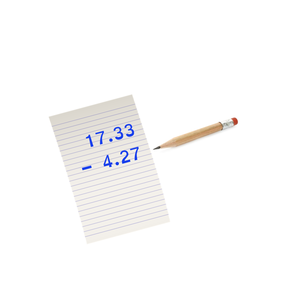Subtracting decimal numbers to 100 using the Standard Algorithm

# Subtracting decimal numbers to 100 using the Standard Algorithm

I can subtract decimals to 100 using the standard algorithm.

No account needed.8,000 schools use Gynzy92,000 teachers use Gynzy1,600,000 students use Gynzy

## General

Students will learn to subtract decimal numbers to 100 using the standard algorithm. They will understand the importance of lining up decimal points and filling the empty spaces after the decimal with zero before they subtract. They will apply these skills to solving word problem

## Standards

CCSS.Math.Content.6.NS.B.3

## Learning objective

Students will be able to subtract decimals to 100 using the standard algorithm.

## Introduction

Students will discuss when they have seen decimals in real life. Examples given are prices at a gas station and times at a swim meet.

## Instruction

Students are instructed on the importance of lining up decimals when copying them. Next, they are shown to fill in missing numbers after the decimal with zeros before they subtract. This is important with subtraction since the zero is often regrouped. They are reminded to place the decimal in the final answer after subtracting. The students will copy several problems on their own to practice lining up and placing decimals in their answers. They are shown that subtracting money amounts includes working with decimals. Further practice is provided with word problems, including some subtracting money amounts. In a final practice activity, they go around a wheel subtracting a series of decimals to get to the final answer

## Quiz

Students respond to ten multiple-choice and true/false questions.

## Closing

Students will be shown a subtraction problem solved on a grid. Students then solve a second problem using a grid.

## Instruction materials

Students are encouraged to copy and solve problems, so paper and pencil is suggested.

### The online teaching platform for interactive whiteboards and displays in schools

• Save time building lessons

• Manage the classroom more efficiently

• Increase student engagement